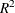The SYSLIN Procedure

PROC SYSLIN Statement

Subsections:
• PROC SYSLIN options;

The following options can be used with the PROC SYSLIN statement.

Data Set Options

DATA=SAS-data-set

specifies the input data set. If the DATA= option is omitted, the most recently created SAS data set is used. In addition to ordinary SAS data sets, PROC SYSLIN can analyze data sets of TYPE=CORR, TYPE=COV, TYPE=UCORR, TYPE=UCOV, and TYPE=SSCP. See the section Special TYPE= Input Data Sets for details.

OUT=SAS-data-set

specifies an output SAS data set for residuals and predicted values. The OUT= option is used in conjunction with the OUTPUT statement. See the section OUT= Data Set for details.

OUTEST=SAS-data-set

writes the parameter estimates to an output data set. See the section OUTEST= Data Set for details.

OUTCOV

COVOUT

writes the covariance matrix of the parameter estimates to the OUTEST= data set in addition to the parameter estimates.

OUTCOV3

COV3OUT

writes covariance matrices for each model in a system to the OUTEST= data set when the 3SLS, SUR, or FIML option is used.

OUTSSCP=SAS-data-set

writes the sum-of-squares-and-crossproducts matrix to an output data set. See the section OUTSSCP= Data Set for details.

Estimation Method Options

2SLS

specifies the two-stage least squares estimation method.

3SLS

specifies the three-stage least squares estimation method.

ALPHA=value

specifies Fuller’s modification to the LIML estimation method. See the section Fuller’s Modification to LIML for details.

CONVERGE=value

specifies the convergence criterion for the iterative estimation methods IT3SLS, ITSUR, and FIML. The default is CONVERGE=0.0001.

FIML

specifies the full information maximum likelihood estimation method.

ITSUR

specifies the iterative seemingly unrelated estimation method.

IT3SLS

specifies the iterative three-stage least squares estimation method.

K=value

specifies the K-class estimation method.

LIML

specifies the limited information maximum likelihood estimation method.

MAXITER=n

specifies the maximum number of iterations allowed for the IT3SLS, ITSUR, and FIML estimation methods. The MAXITER= option can be abbreviated as MAXIT=. The default is MAXITER=30.

MELO

specifies the minimum expected loss estimation method.

NOINCLUDE

excludes the RESTRICT statements from the final stage for the 3SLS, IT3SLS, SUR, and ITSUR estimation methods.

OLS

specifies the ordinary least squares estimation method. This is the default.

SDIAG

uses the diagonal of S instead of S to do the estimation, where S is the covariance matrix of equation errors. See the section Uncorrelated Errors across Equations for details.

SINGULAR=value

specifies a criterion for testing singularity of the crossproducts matrix. This is a tuning parameter used to make PROC SYSLIN more or less sensitive to singularities. The value must be between 0 and 1. The default is SINGULAR=1E–8.

SUR

specifies the seemingly unrelated estimation method.

Printing Control Options

ALL

specifies the CORRB, COVB, DW, I, OVERID, PLOT, STB, and XPX options for every MODEL statement.

FIRST

prints first-stage regression statistics for the endogenous variables regressed on the instruments. This output includes sums of squares, estimates, variances, and standard deviations.

ITPRINT

prints parameter estimates, system-weighted residual sum of squares, andat each iteration for the IT3SLS and ITSUR estimation methods. For the FIML method, the ITPRINT option prints parameter estimates, negative of log-likelihood function, and norm of gradient vector at each iteration.

NOPRINT

suppresses all printed output. Specifying NOPRINT in the PROC SYSLIN statement is equivalent to specifying NOPRINT in every MODEL statement.

REDUCED

prints the reduced form estimates. If the REDUCED option is specified, you should specify any IDENTITY statements needed to make the system square. See the section Reduced Form Estimates for details.

SIMPLE

prints descriptive statistics for the dependent variables. The statistics printed include the sum, mean, uncorrected sum of squares, variance, and standard deviation.

USSCP

prints the uncorrected sum-of-squares-and-crossproducts matrix.

USSCP2

prints the uncorrected sum-of-squares-and-crossproducts matrix for all variables used in the analysis, including predicted values of variables generated by the procedure.

VARDEF=DF | N | WEIGHT | WGT

specifies the denominator to use in calculating cross-equation error covariances and parameter standard errors and covariances. The default is VARDEF=DF, which corrects for model degrees of freedom. VARDEF=N specifies no degrees-of-freedom correction. VARDEF=WEIGHT specifies the sum of the observation weights. VARDEF=WGT specifies the sum of the observation weights minus the model degrees of freedom. See the section Computation of Standard Errors for details.